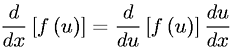Equations > Calculus > Differentiation/Differentals > Chain Rule of Differentiation

### Chain Rule of DifferentiationLatex Code:

MathML Code:

 $\fracd\mathrm{dx}\left[f\left(u\right)\right]=\fracd\mathrm{du}\left[f\left(u\right)\right]\frac\mathrm{du}\mathrm{dx}$

MathType 5.0: